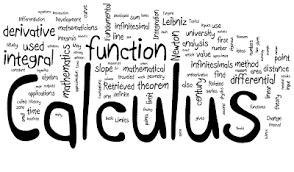#### CALCULUS-II

Calculus, branch of mathematics concerned with the calculation of instantaneous rates of change (differential calculus) and the summation of infinitely many small factors to determine some whole (integral calculus). Two mathematicians, Isaac Newton of England and Gottfried Wilhelm Leibniz of Germany, share credit for having independently developed the calculus in the 17th century. Calculus is now the basic entry point for anyone wishing to study physics, chemistry etc.